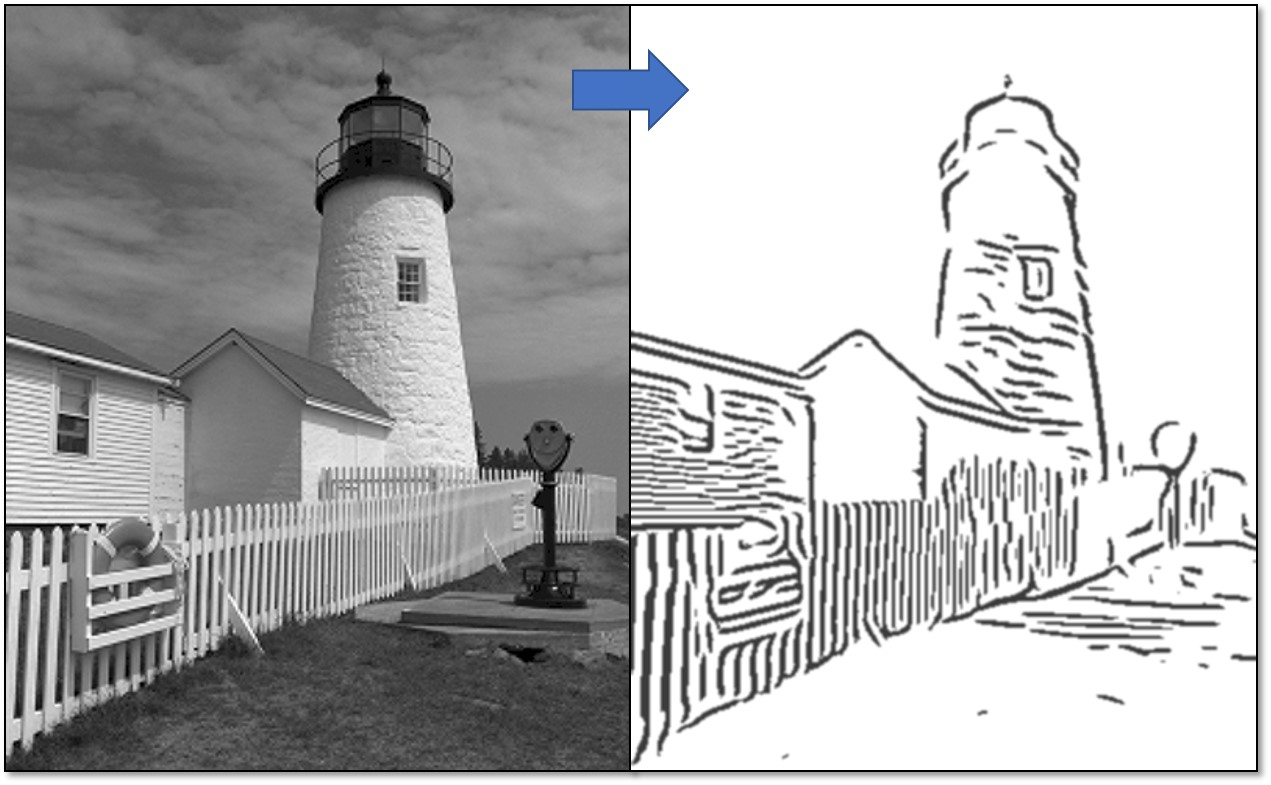輸入一張影像，即可產生出一張線條藝術風格畫。

Implementation

1. 由輸入影像產生邊緣向量流場
2. 反覆的精煉、平滑化邊緣向量流場
3. 藉由邊緣向量流場的資訊，對原圖套用高斯差以產生特徵線條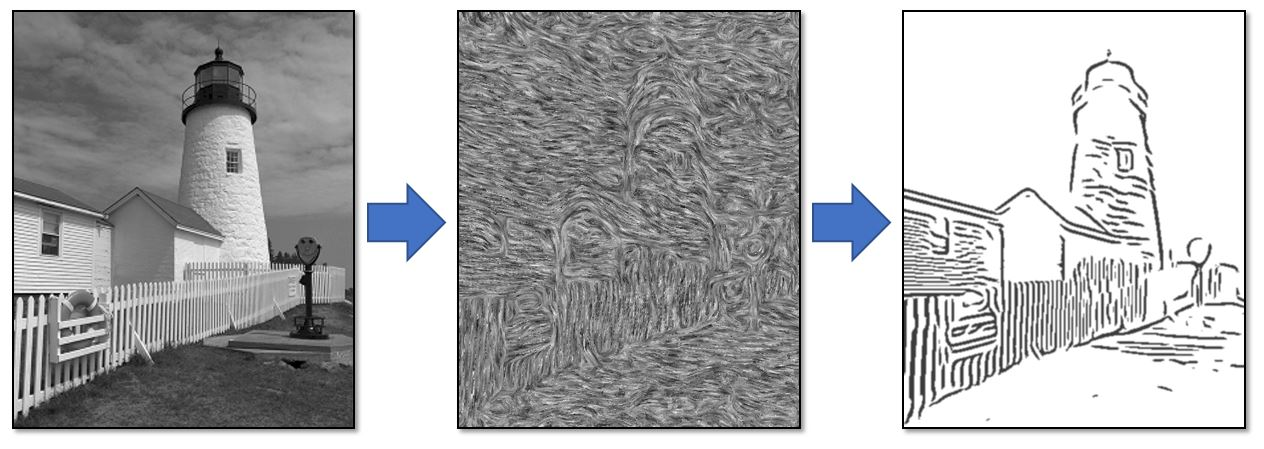整個方法的流程圖： 由原圖產生邊緣向量流場，再藉由流場資訊套用高斯差產生線條畫。

Edge Tangent Flow

1. 可以描述重要邊緣的流向。
2. 鄰近的向量必須要平滑(避免鄰近的向量方向差太多)，除非是角落。
3. 重要的邊緣必須要維持它原本的方向。

Generate initial ETF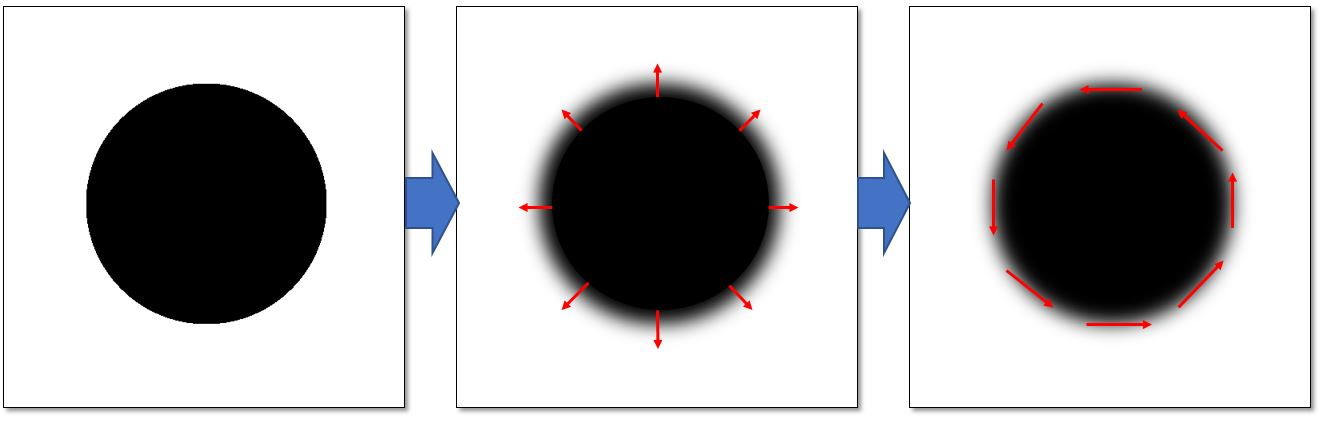對原圖做適當模糊後即可取得灰階梯度向量(GVF)，再旋轉 90 度可得一個初始的 ETF。

Refining ETF

$$t^{new}=\dfrac {1} {k}\sum _{y\in \Omega \left( x\right) }\phi \left( x,y\right) t^{cur}\left(y\right)w_{s}\left( x,y\right)w_{m}\left( x,y\right)w_{d}\left( x,y\right)$$

$$w_{s}\left( x,y\right)$$ 是一個圓形 box filter function，落在外面的向量權重為零
$$w_{m}\left( x,y\right)$$ 為 magnitude weight function，用於確保重要的邊緣方向會被保留
$$w_{d}\left( x,y\right)$$ 為 direction weight function，用於使得鄰居的向量方向不會差距過大
$$\phi \left( x,y\right)$$ 則是當兩向量夾角過大時會反轉方向以確保夾角不會大於 90 度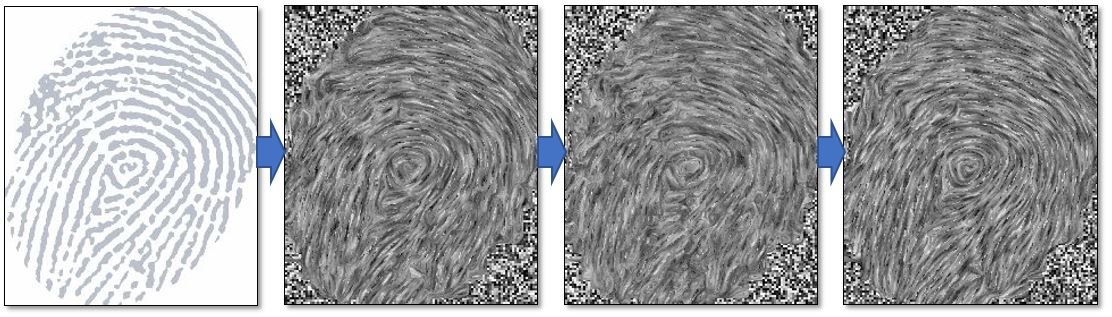由左至右：原圖、用 GVF 得到之初始 ETF、經過一次平滑化、經過兩次平滑化。 kernel size=7

Line construction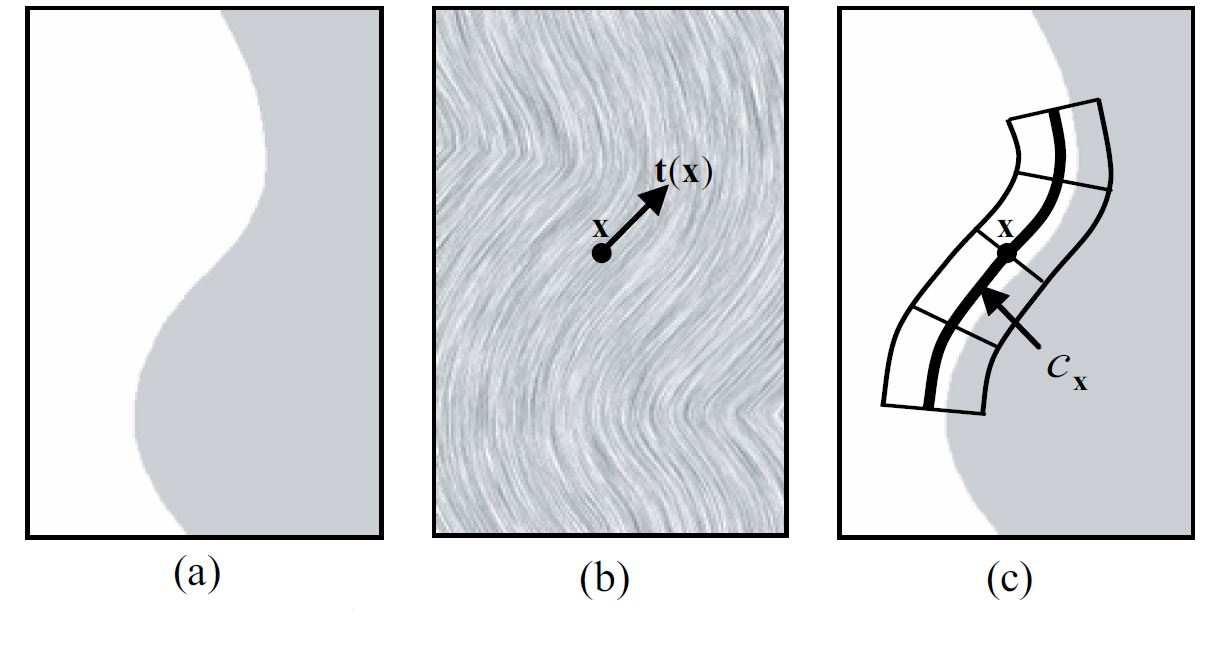(a)原圖、(b)ETF、(c)沿著流場的 kernel。

Flow-based Difference-of-Gaussians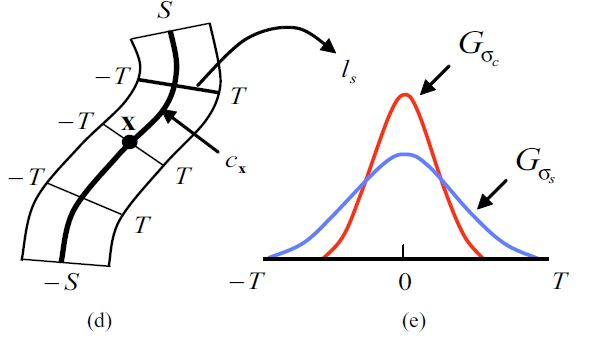(d)kernel 詳細圖示、(e)高斯差示意圖。

$$F\left( s\right) =\int _{-T}^{T}I\left( l_{s}\left( t\right) \right) f\left( t\right) dt$$

$$G\left( x\right) =\int _{-S}^{S}G_{\sigma}\left( s \right) F\left( s\right) ds$$

Iterative FDoG filtering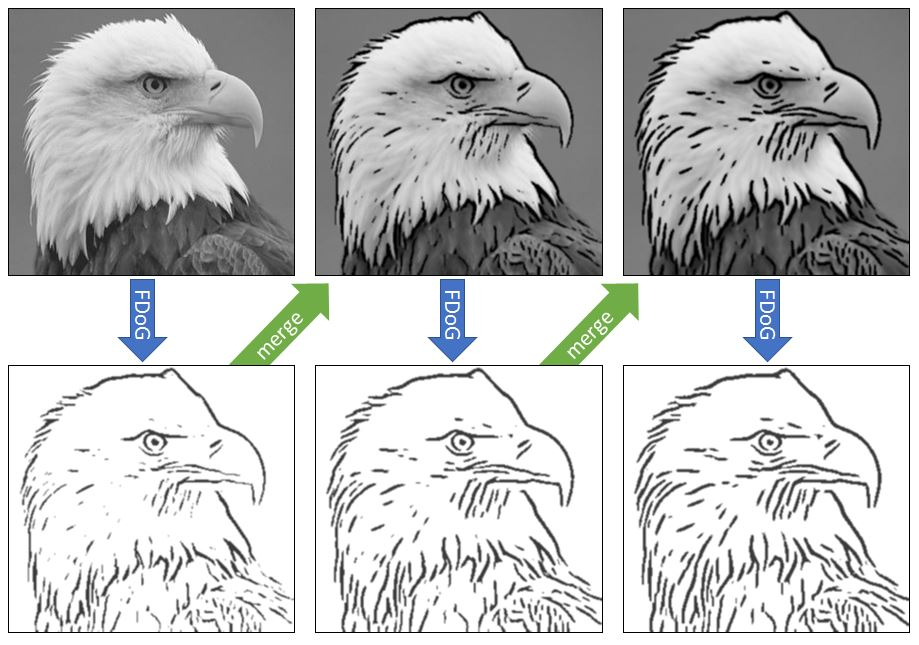藉由將結果疊合回原圖再做一次 FDoG，可以使的結果品質越來越好。

Results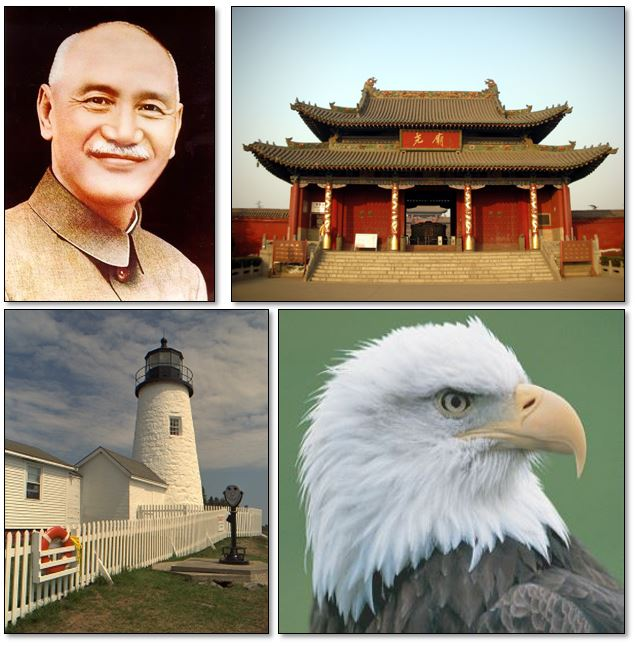輸入影像。由左上至右下：蔣公、廟、燈塔、老鷹。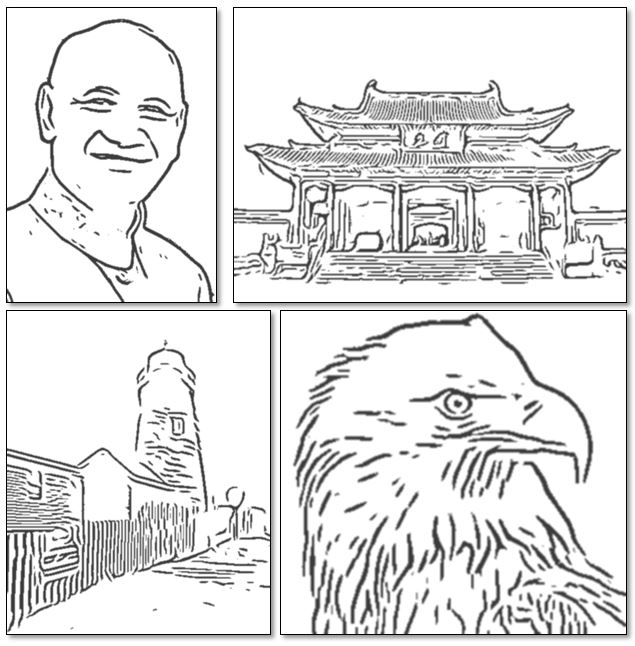輸出結果。由左上至右下：蔣公、廟、燈塔、老鷹。

Source code

You can find my implementation source code at github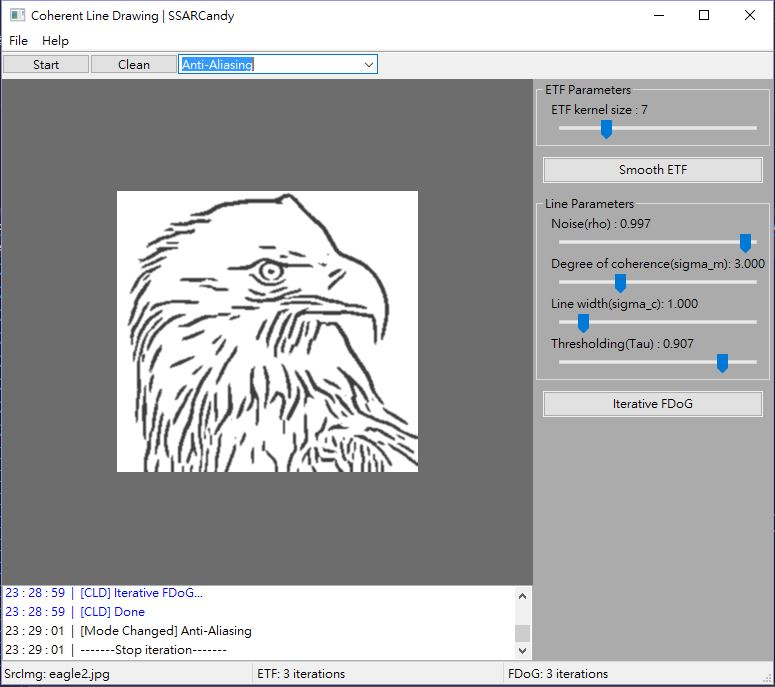Screenshot of my system user interface